# RS Aggarwal Solutions for Class 8 Maths Chapter 24 - Pie Charts Exercise 24A

Students can refer and download RS Aggarwal Solutions for Class 8 Maths Chapter 24- Exercise 24A, Pie Charts from the links provided below. RS Aggarwal Solutions provides answers in a comprehensive way to help students understand the concepts in a better way. The explanations for each problem presented by the faculties are clear cut and simple. In Exercise 24A of RS Aggarwal Class 8 Maths, we see questions based on the Construction of Pie Charts.

## Download PDF of RS Aggarwal Solutions for Class 8 Maths Chapter 24- Pie Charts Exercise 24A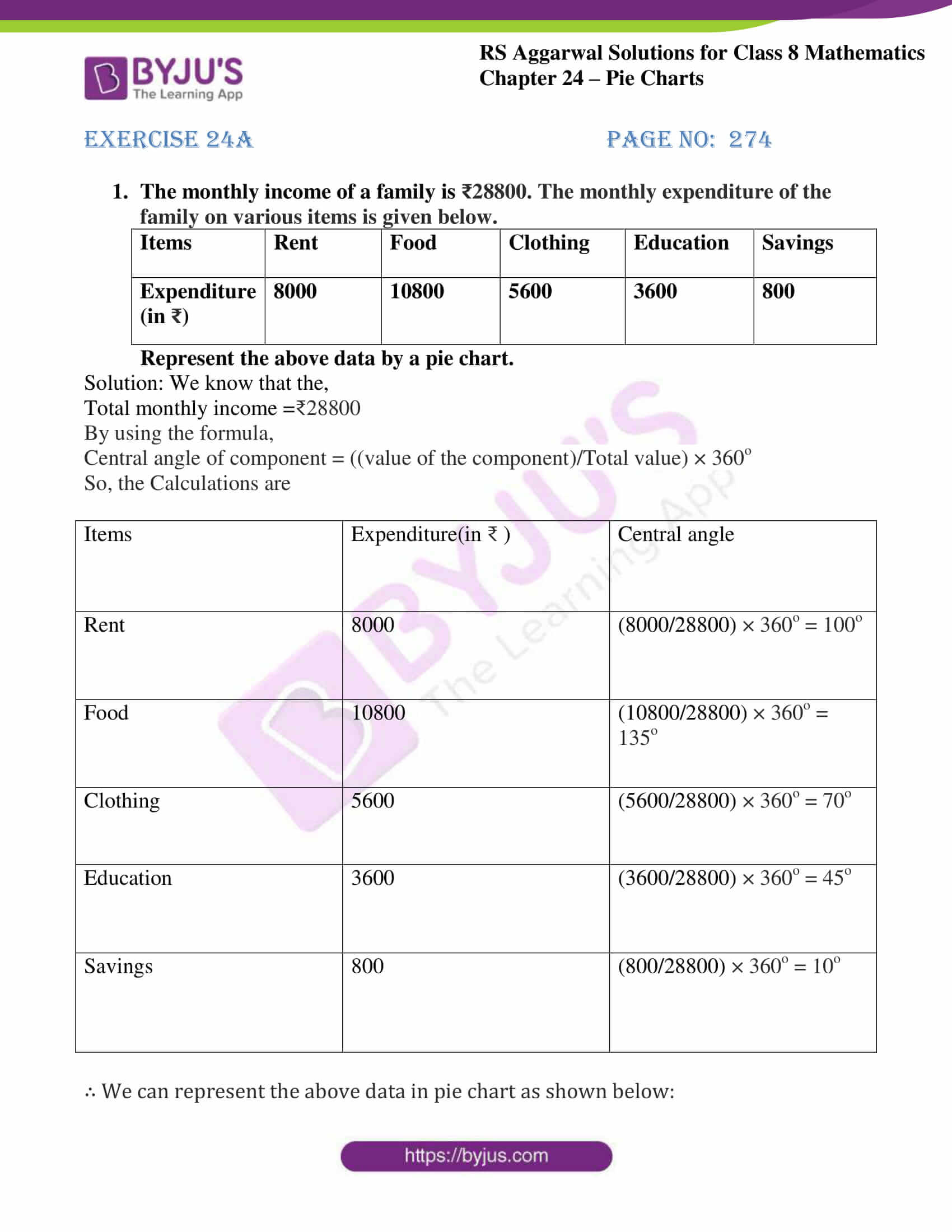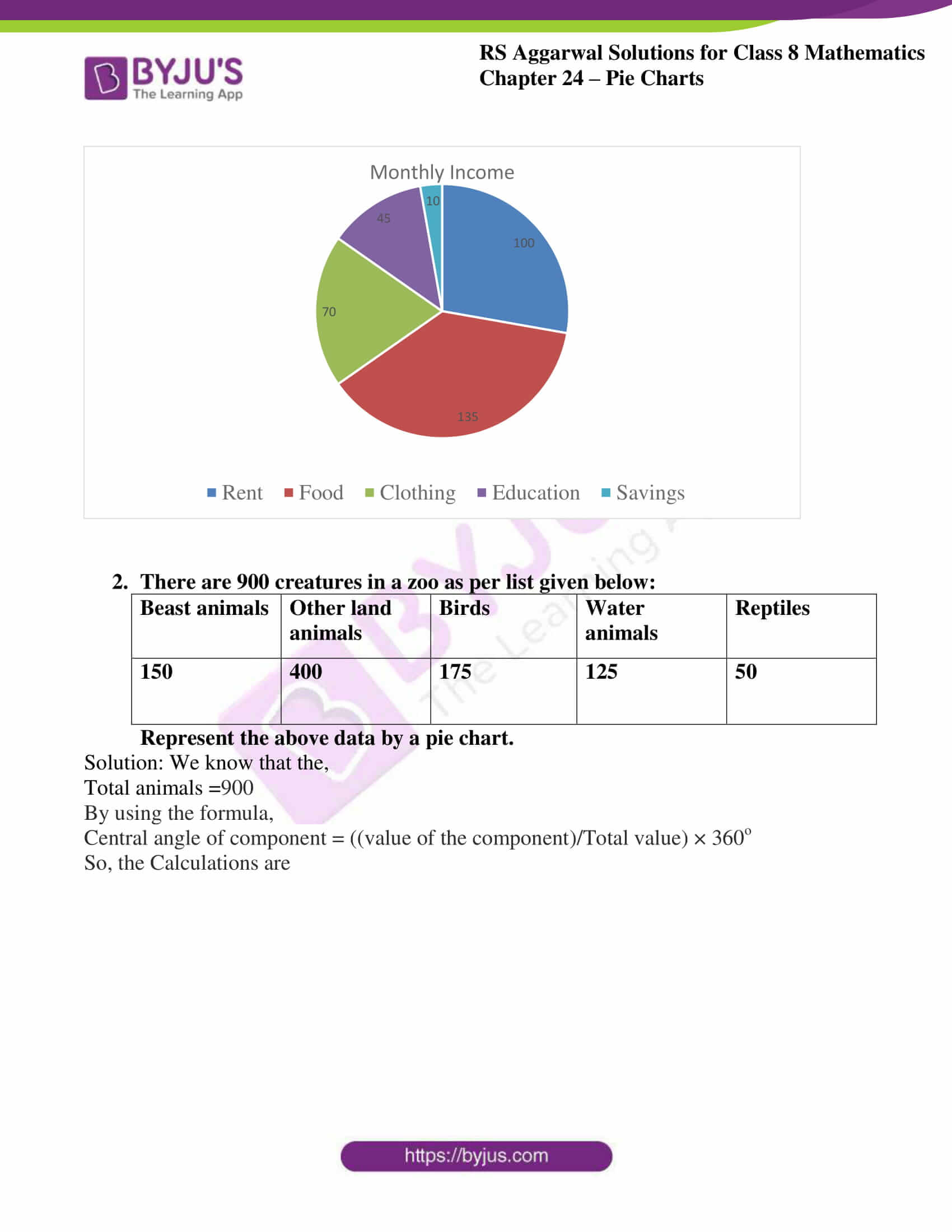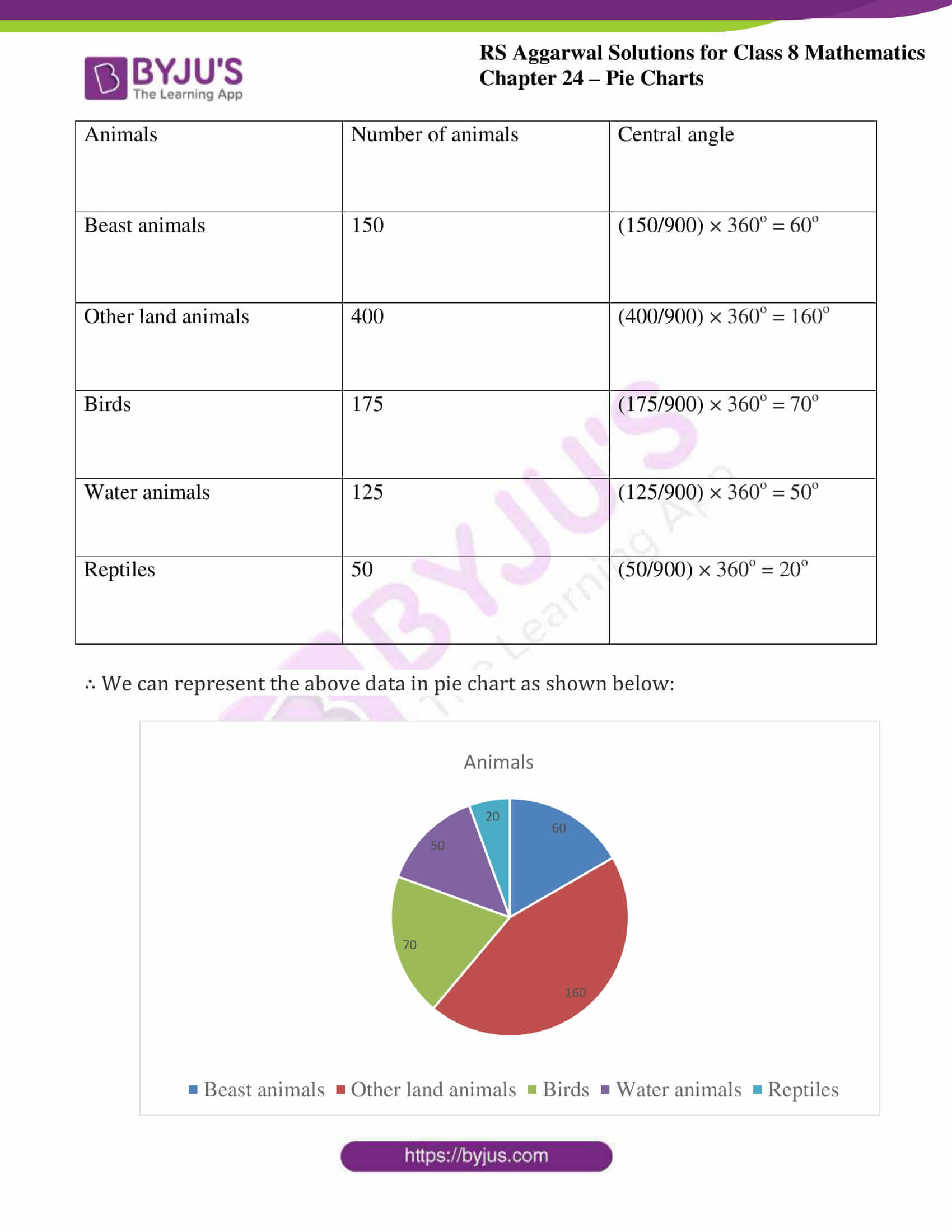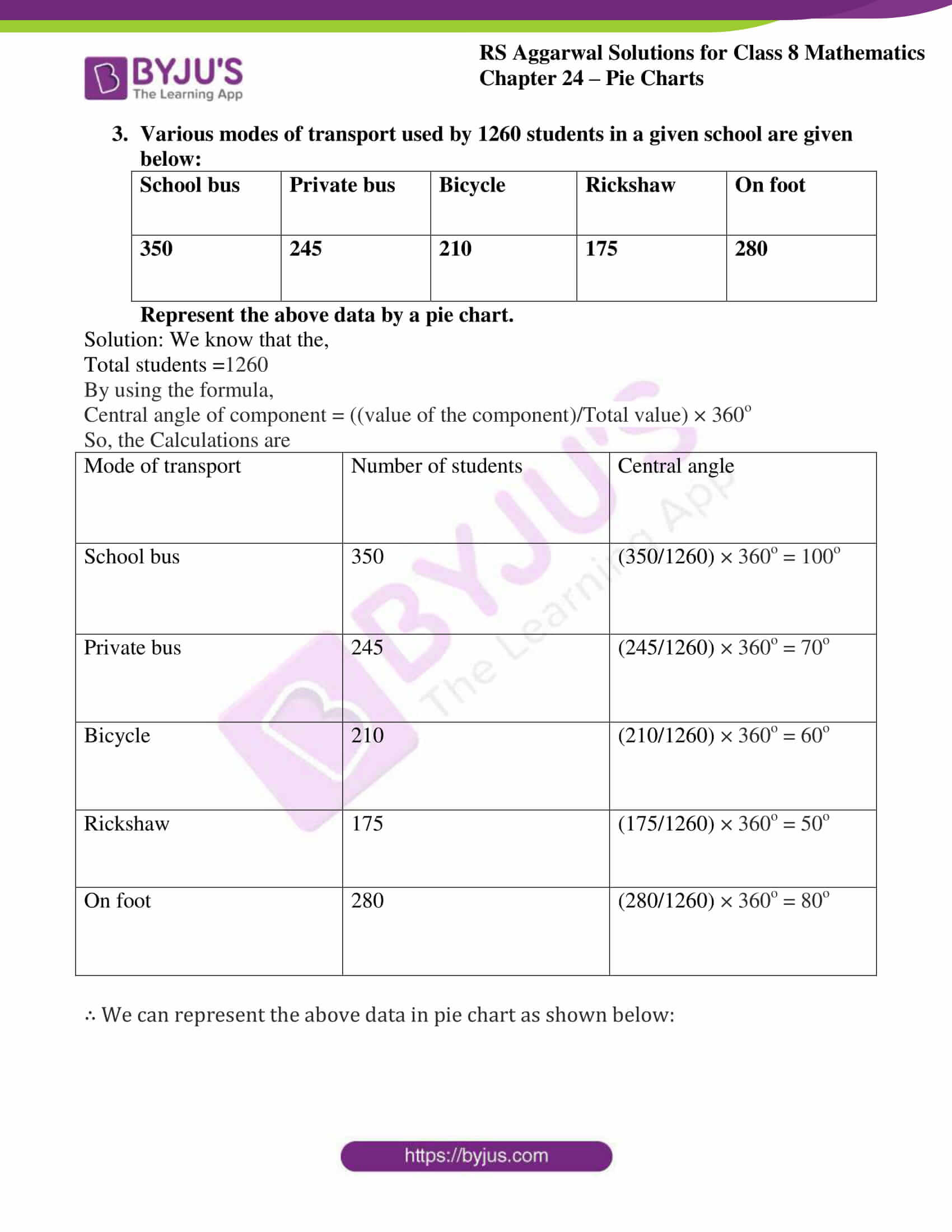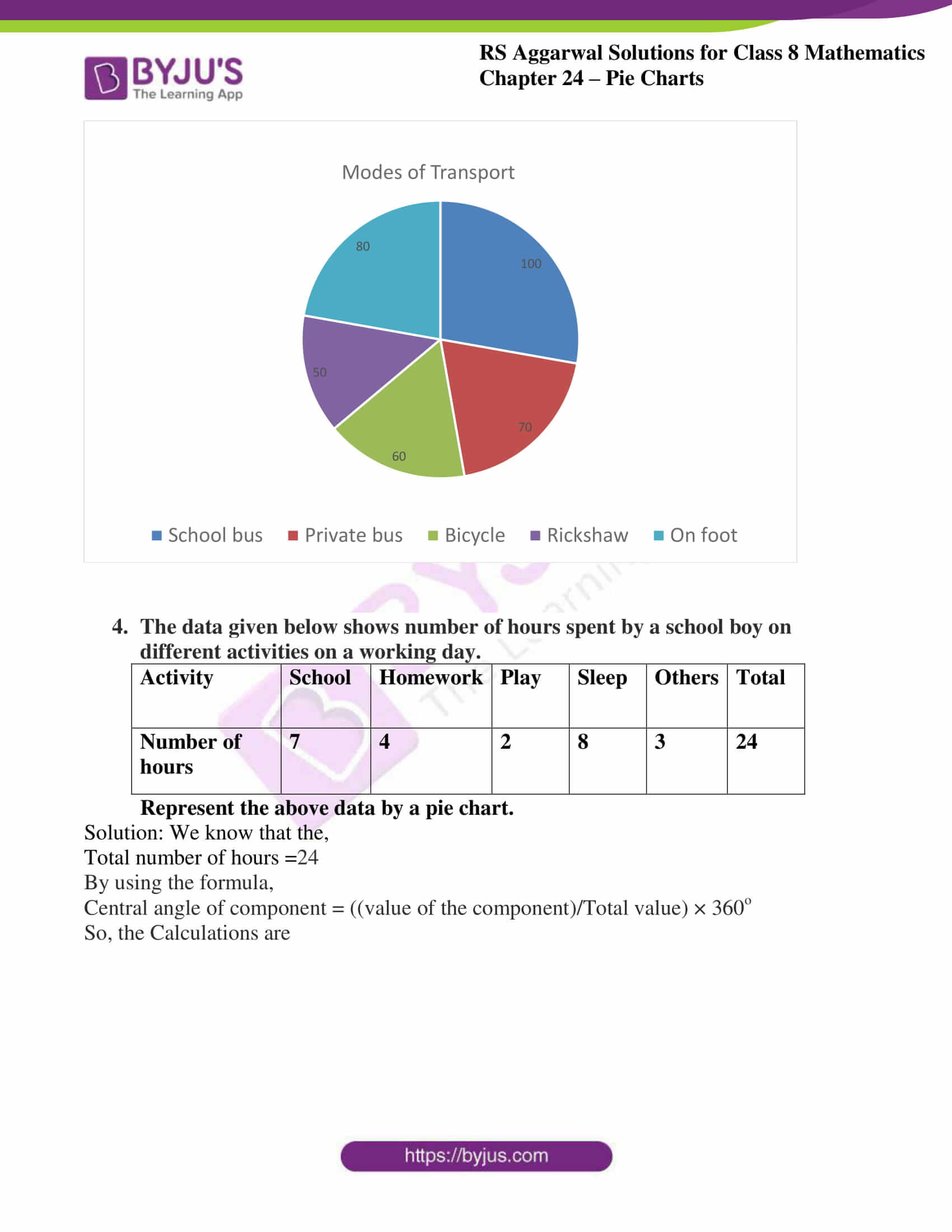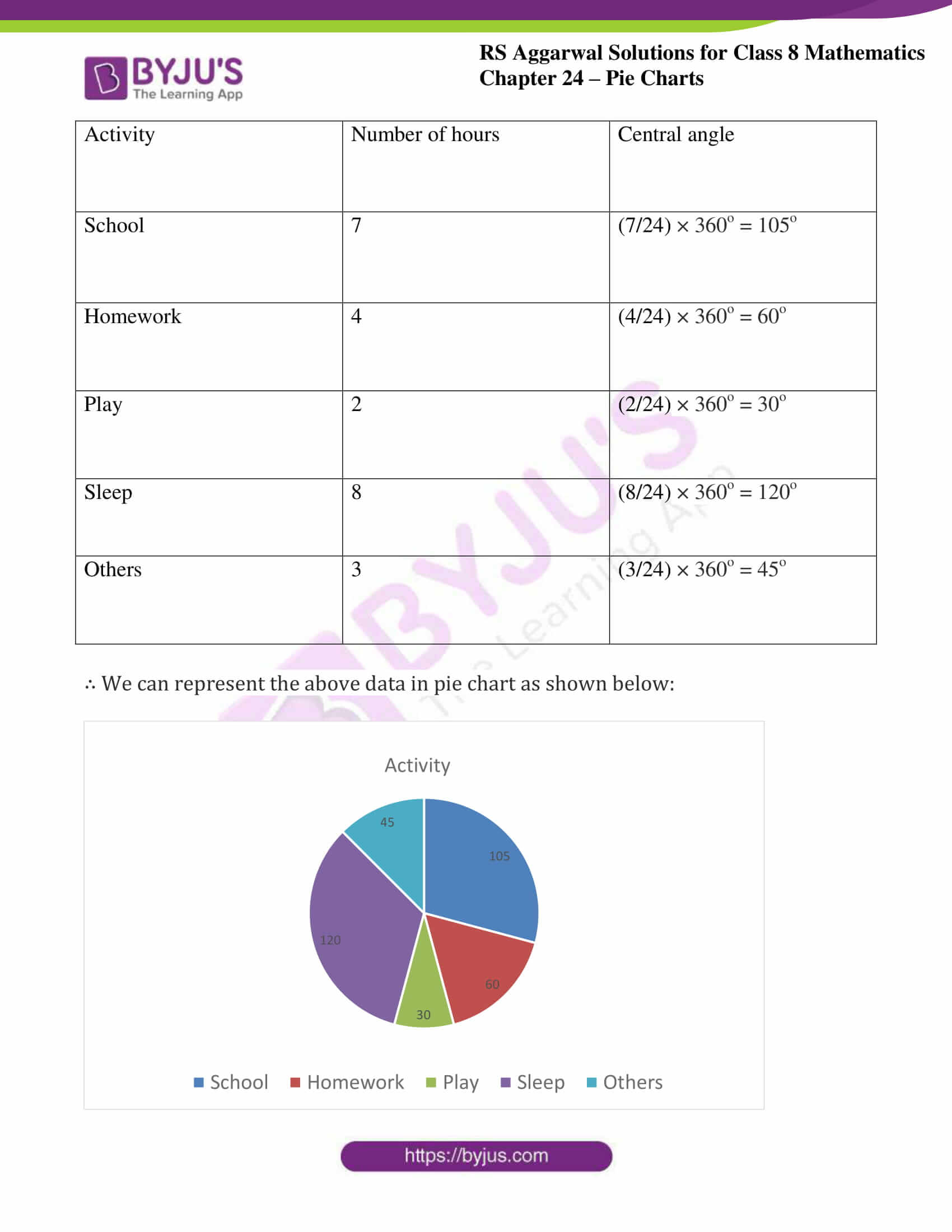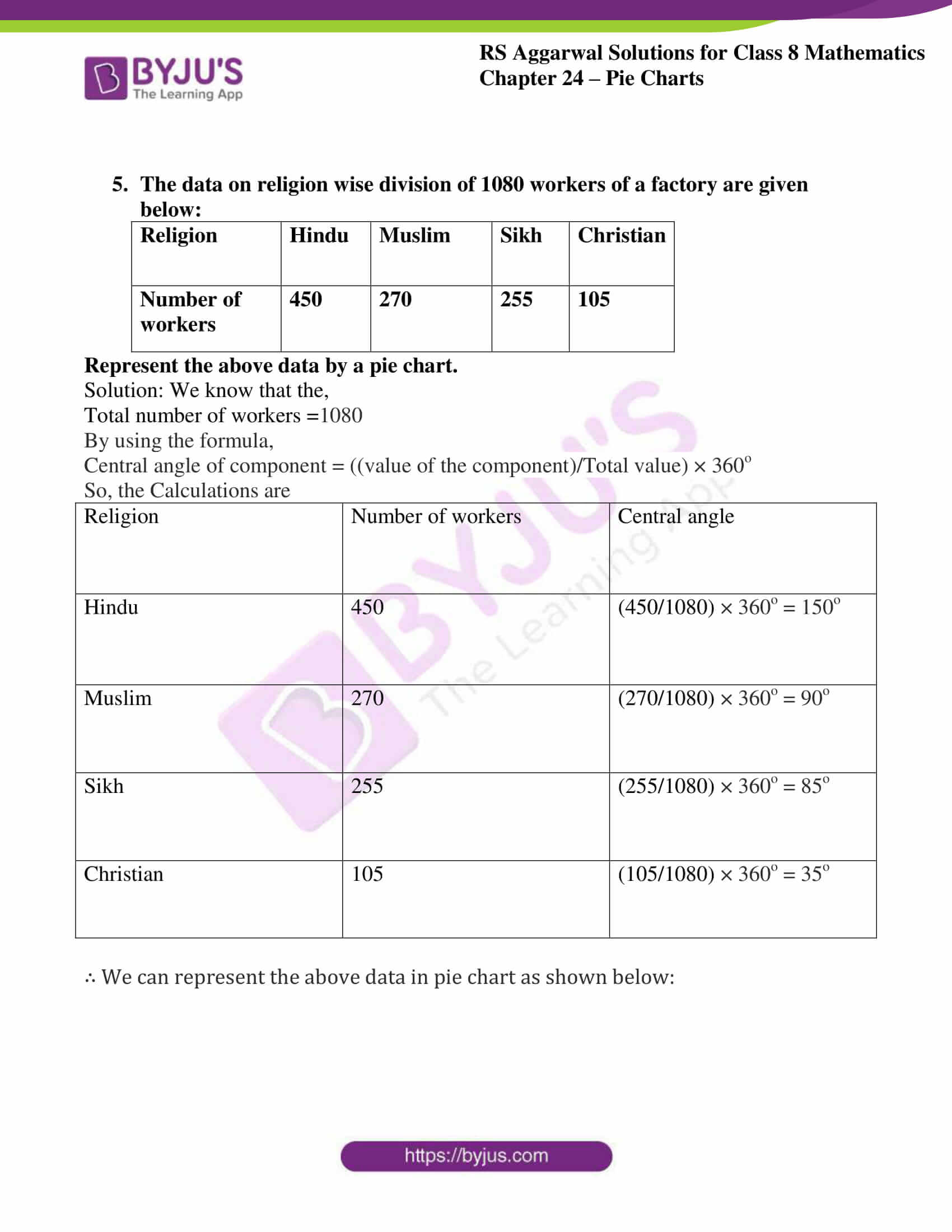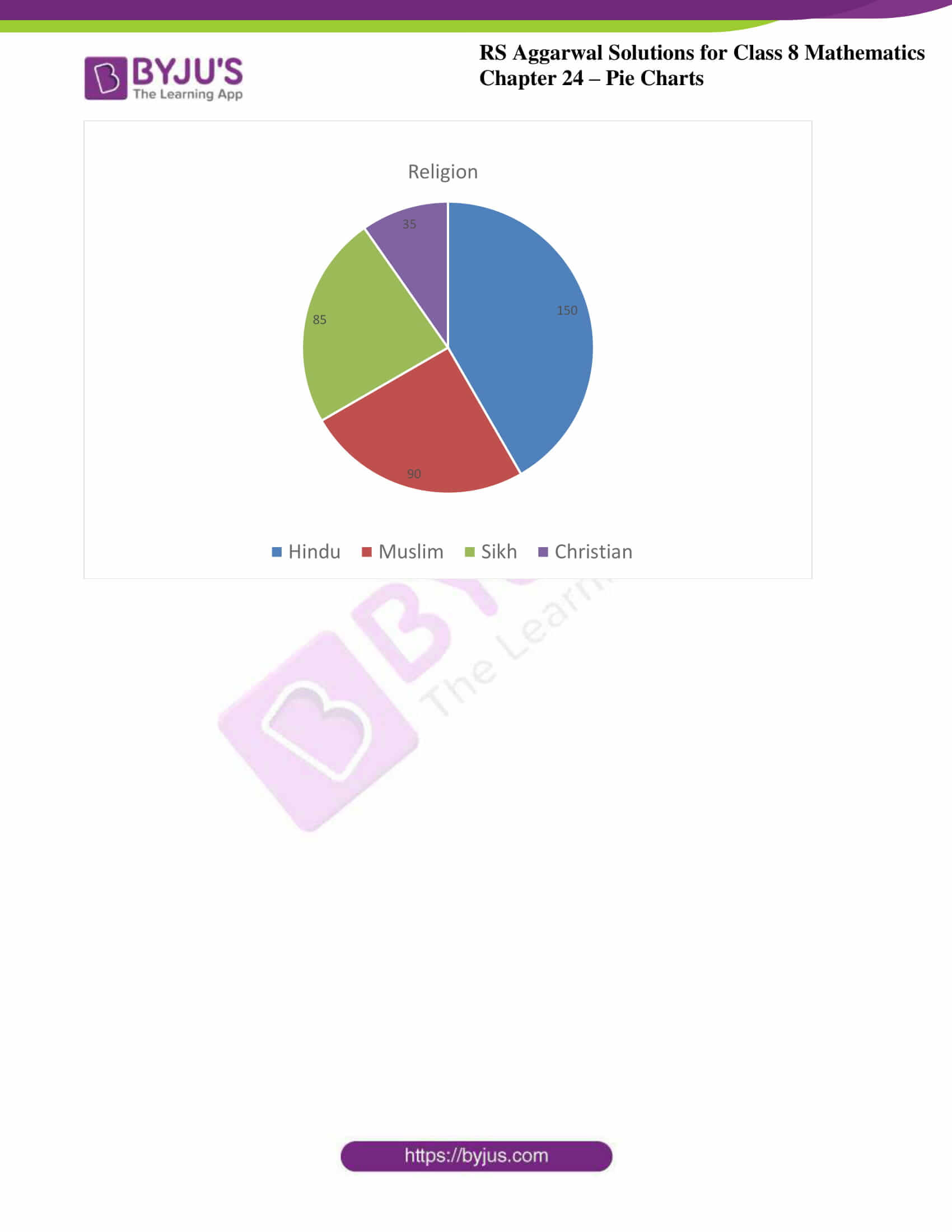### Access Answers to RS Aggarwal Solutions for Class 8 Maths Chapter 24- Pie Charts Exercise 24A

1. The monthly income of a family is ₹28800. The monthly expenditure of the family on various items is given below.

 Items Rent Food Clothing Education Savings Expenditure (in ₹) 8000 10800 5600 3600 800

Represent the above data by a pie chart.
Solution: We know that the,
Total monthly income = ₹28800
By using the formula,
Central angle of component = ((value of the component)/Total value) × 360o
So, the Calculations are

 Items Expenditure(in ₹ ) Central angle Rent 8000 (8000/28800) × 360o = 100o Food 10800 (10800/28800) × 360o = 135o Clothing 5600 (5600/28800) × 360o = 70o Education 3600 (3600/28800) × 360o = 45o Savings 800 (800/28800) × 360o = 10o

∴ We can represent the above data in pie chart as shown below: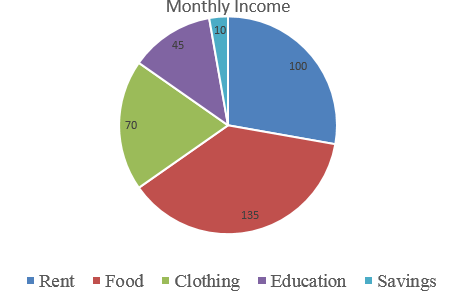2. There are 900 creatures in a zoo as per list given below:

 Beast animals Other land animals Birds Water animals Reptiles 150 400 175 125 50

Represent the above data by a pie chart.
Solution: We know that the,
Total animals =900
By using the formula,
Central angle of component = ((value of the component)/Total value) × 360o
So, the Calculations are

 Animals Number of animals Central angle Beast animals 150 (150/900) × 360o = 60o Other land animals 400 (400/900) × 360o = 160o Birds 175 (175/900) × 360o = 70o Water animals 125 (125/900) × 360o = 50o Reptiles 50 (50/900) × 360o = 20o

∴ We can represent the above data in pie chart as shown below: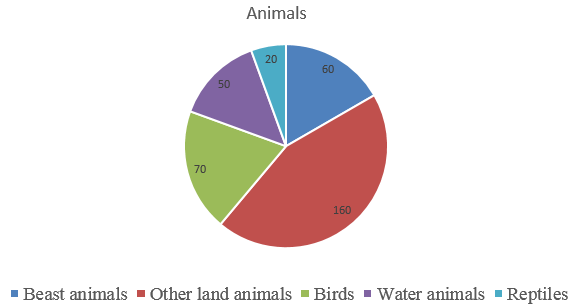3. Various modes of transport used by 1260 students in a given school are given below:

 School bus Private bus Bicycle Rickshaw On foot 350 245 210 175 280

Represent the above data by a pie chart.
Solution: We know that the,
Total students =1260
By using the formula,
Central angle of component = ((value of the component)/Total value) × 360o
So, the Calculations are

 Mode of transport Number of students Central angle School bus 350 (350/1260) × 360o = 100o Private bus 245 (245/1260) × 360o = 70o Bicycle 210 (210/1260) × 360o = 60o Rickshaw 175 (175/1260) × 360o = 50o On foot 280 (280/1260) × 360o = 80o

∴ We can represent the above data in pie chart as shown below: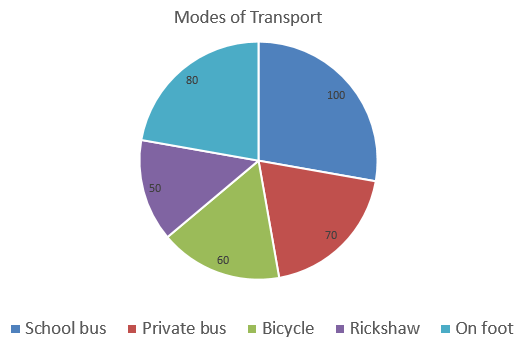4. The data given below shows number of hours spent by a school boy on different activities on a working day.

 Activity School Homework Play Sleep Others Total Number of hours 7 4 2 8 3 24

Represent the above data by a pie chart.
Solution: We know that the,
Total number of hours =24
By using the formula,
Central angle of component = ((value of the component)/Total value) × 360o
So, the Calculations are

 Activity Number of hours Central angle School 7 (7/24) × 360o = 105o Homework 4 (4/24) × 360o = 60o Play 2 (2/24) × 360o = 30o Sleep 8 (8/24) × 360o = 120o Others 3 (3/24) × 360o = 45o

∴ We can represent the above data in pie chart as shown below: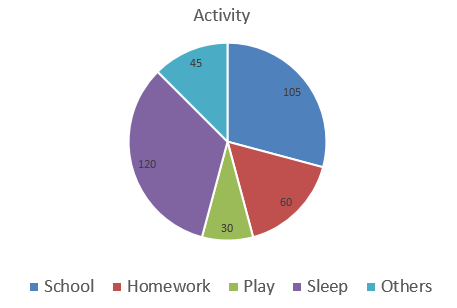5. The data on religion wise division of 1080 workers of a factory are given below:

 Religion Hindu Muslim Sikh Christian Number of workers 450 270 255 105

Represent the above data by a pie chart.
Solution: We know that the,
Total number of workers =1080
By using the formula,
Central angle of component = ((value of the component)/Total value) × 360o
So, the Calculations are

 Religion Number of workers Central angle Hindu 450 (450/1080) × 360o = 150o Muslim 270 (270/1080) × 360o = 90o Sikh 255 (255/1080) × 360o = 85o Christian 105 (105/1080) × 360o = 35o

∴ We can represent the above data in pie chart as shown below: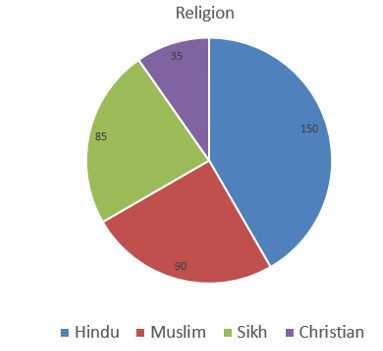### Access other Exercise of RS Aggarwal Solutions for Class 8 Maths Chapter 24- Pie Charts

Exercise 24B Solutions 4 Questions

## RS Aggarwal Solutions for Class 8 Maths Chapter 24- Pie Charts Exercise 24A

Exercise 24A of RS Aggarwal Class 8, Pie Charts, mainly consists of questions related to the Construction of Pie Charts by calculating the central angle for a component.

The RS Aggarwal Solutions help the students to answer the questions from the textbook without any difficulty. Students are suggested to practice the problems regularly which will help them excel in their exams and increase their overall percentage. Practising as many times as possible helps in building time management skills and also boosts the confidence level to achieve high marks.